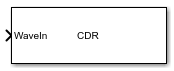# CDR

Models a clock data recovery circuit

• Library:
• SerDes Toolbox / Datapath Blocks

•## Description

The CDR block provides clock sampling times and estimates data symbols at the receiver using a first order phase tracking CDR model. For more information, see Clock and Data Recovery in SerDes System..

## Ports

### Input

expand all

Input baseband signal. The input to the CDR must be applied as one sample at a time and not as a vector.

Data Types: `double`

## Parameters

expand all

Clock phase offset, specified as a real scalar in the range [0, 0.5] in fraction of symbol time. Phase offset manually shifts clock probability distribution function (PDF) for better bit error rate (BER).

#### Programmatic Use

• Use `get_param(gcb,'PhaseOffset')` to view the current value of Phase offset (symbol time).

• Use `set_param(gcb,'PhaseOffset',value)` to set CDR to a specific Phase offset (symbol time).

Data Types: `double`

Reference clock offset impairment, specified as a real scalar in the range [0, 300] in parts per million (ppm). Reference offset (ppm) is the deviation between transmitter oscillator frequency and receiver oscillator frequency.

#### Programmatic Use

• Use `get_param(gcb,'ReferenceOffset')` to view the current value of Reference offset (ppm).

• Use `set_param(gcb,'ReferenceOffset',value)` to set CDR to a specific Reference offset (ppm).

Data Types: `double`

Early or late CDR count threshold to trigger a phase update, specified as a unitless real positive integer ≥5. Increasing the value of Early/late count threshold provides a more stable output clock phase at the expense of convergence speed. Because the bit decisions are made at the clock phase output, a more stable clock phase has a better bit error rate (BER).

Early/late count threshold also controls the bandwidth of the CDR which is approximately calculated by using the equation:

#### Programmatic Use

• Use `get_param(gcb,'Count')` to view the current value of Early/late count threshold.

• Use `set_param(gcb,'Count',value)` to set CDR to a specific Early/late count threshold.

Data Types: `double`

Clock phase resolution, specified as a real scalar in fraction of symbol time. Step (symbol time) is the inverse of the number of phase adjustments in CDR.

#### Programmatic Use

• Use `get_param(gcb,'Step')` to view the current value of Sensitivity.

• Use `set_param(gcb,'Step',value)` to set CDR to a specific Sensitivity.

Data Types: `double`

Sampling latch metastability voltage, specified as a real scalar in volts. If the data sample voltage lies within the region (±Sensitivity (V)), there is a 50% probability of bit error.

#### Programmatic Use

• Use `get_param(gcb,'Sensitivity')` to view the current value of Sensitivity (V).

• Use `set_param(gcb,'Sensitivity',value)` to set CDR to a specific Sensitivity (V).

Data Types: `double`

IBIS-AMI parameters

Select to include Phase Offset as a parameter in the IBIS-AMI file. If you deselect Phase Offset, it is removed from the AMI files, effectively hard-coding Phase Offset to its current value.

Select to include Reference offset as a parameter in the IBIS-AMI file. If you deselect Reference offset, it is removed from the AMI files, effectively hard-coding Reference offset to its current value.

## Version History

Introduced in R2019a